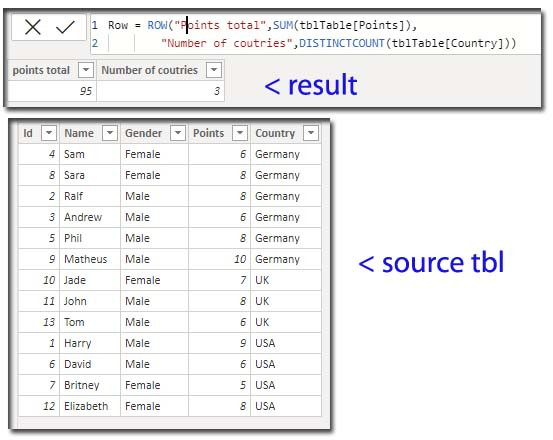Search
• Marek Vavrovic

# SELECTCOLUMNS, ROW

Updated: Oct 5

ROW function.

Returns a table with a single row containing values that result from the expressions given to each column.

Syntax

ROW(<name>, <expression>[[,<name>, <expression>]…])

Parameters

Term Definition

name The name of the column, enclosed in double quotes.

expression Any DAX expression that returns a single scalar value to populate name.

Example 1:

This formula returns total number of points and number of countries.Example 2

Row =

ROW (

"Points total", SUM ( tblTable[Points] ),

"Number of countries", DISTINCTCOUNT ( tblTable[Country] )

),

"Ratio", DIVIDE ( [Number of countries], [points total] )

)Example 3

with UNION

syntax

UNION(<table_expression1>, <table_expression2> [,<table_expression>]…)## SELECTCOLUMNS

This function returns the selected columns from some source table.

Syntax:

SELECTCOLUMNS(<table>, <name>, <scalar_expression>)

Table manipulation functions are not supported for use in DirectQuery mode.

This function doesn't keep the source table columnsOne big difference of SelectColumns and AddColumns is that AddColumns keep all the existing columns in the table and adds more columns to that, But the SelectColumns starts with no columns from the table, and builds a custom subset of columns or with additional calculated columns on it.## Recent Posts

See All

#### Index

Information functions Filter functions Table manipulation functions Grouping and summarizing Time intelligence functions OPENINGBALANCEYEAR OPENINGBALANCEYEAR(<Expression>,<Dates> [,<Filter>][,<YearEn